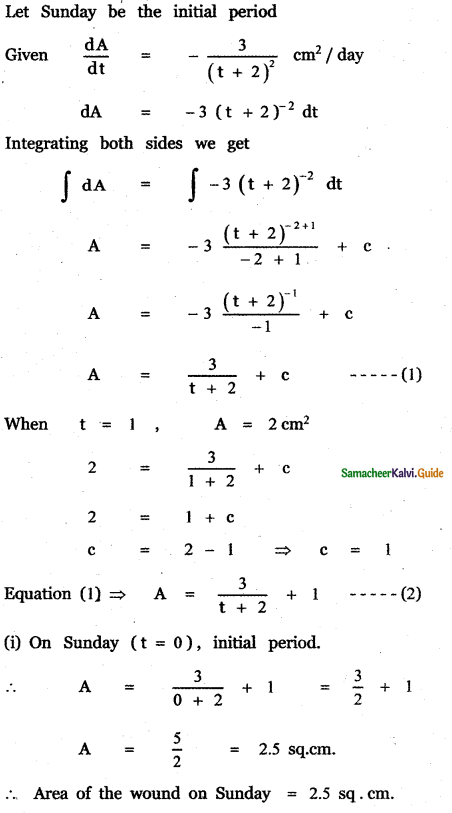Tamilnadu State Board New Syllabus Samacheer Kalvi 11th Maths Guide Pdf Chapter 11 Integral Calculus Ex 11.4 Text Book Back Questions and Answers, Notes.

## Tamilnadu Samacheer Kalvi 11th Maths Solutions Chapter 11 Integral Calculus Ex 11.4

Question 1.
If f'(x) = 4x – 5 and f(2) = 1, find f(x)
$$\int f^{\prime}(x) d x=\int(4 x-5) d x$$
f(x) = $$\frac{4 x^{2}}{2}$$ – 5x + c
f(x) = 2x2 – 5x + c
But f(2) = 1
2(2)2 – 5(2) + c = 1
8 – 10 + c = 1
c = 3
Thus, f(x) = 2x2 – 5x + 3Question 2.
If f'(x) = 9x2 – 6x and f(0) = – 3 find f(x).
Given f’ (x) = 9x2 – 6x and f(0) = – 3
$$\frac{\mathrm{d} f(x)}{\mathrm{d} x}$$ = 9x2 – 6x
df(x) = (9x2 – 6x) dx
∫ df(x) = ∫ (9x2 – 6x)
∫ df(x) = ∫ 9x2 dx – ∫ 6x dx
f(x) = 9 ∫x2 dx – 6 ∫ x dx
f(x) = 9 × $$\frac{x^{3}}{3}$$ – 6 $$\frac{x^{2}}{2}$$ + C
f(x) = 3x3 – 3x2 + c —— (1)
f(o) = – 3
(1) ⇒ f(0) = 3 × – 3 . 02 + c
– 3 = 0 – 0 ⇒c = – 3
Substituting in equation (1) we get
f(x) = 3x3 – 3x2 – 3
f(x) = 3(x3 – x2 – 1)Question 3.
If f” (x) = 12x – 6 and f(1) = 30, f’ (1) = 5, find f(x)

f'(x) =  – 6x + c
f'(x) = 6x2 – 6x + c
But f'(1) = 5
6(1)2 – 6(1) + c = 5
c = 5
f” (x) = 6x2 – 6x + 5
$$\int f^{\prime \prime}(x) d x=\int\left(6 x^{2}-6 x+5\right) d x$$
f(x) = $$\frac{6 x^{3}}{3}-\frac{6 x^{2}}{2}$$ + 5x + c
f(x) = 2x3 – 3x2 + 5x + c
But f(1) = 30
2(1)3 – 3(1)2 + 5(1) + c = 30
2 – 3 + 5 + c = 30
c = 26
f(x) = 2x3 – 3x2 + 5x + 26Question 4.
A ball is thrown vertically upward from the ground with an initial velocity of 39.2 m / sec. If the only force considered is that attributed to the acceleration due to gravity, find
(i) how long will it take for the ball to strike the ground?
(ii) the speed with which will it strike the ground? and
(iii) how high the ball will rise?
Initial velocity of the ball = 39.2 in / s
Let s be the distance of the ball from the ground at time t.
u = 39.2m/s.
s = ut – $$\frac{1}{2}$$ . gt2 where g = 9.8 m / sec.
s = 39.2 t – $$\frac{1}{2}$$ × (9.8) t2
s = 39.2 t – 49 t2

(i) how long will it take for the ball to strike the ground?
For the ball to strike the ground, s = 0
∴ 39.2 t – 4.9 t2 = 0
t(39.2 – 4.9 t) = 0
39.2 – 4.9 t = 0 since t ≠ 0
4.9 t = 39.2
t = $$\frac{39.2}{4.9}$$ = 8
t = 8 sec.(ii) The speed with which will it strike the ground?
At t = 8; (1) ⇒ v = -9.8(8) + 39.2
= -78.4 + 39.2
= -39.2
∴ The speed with which the ball will strike the ground is = 39.2 m/s
(iii) At maximum height v = 0
⇒ (2) ⇒ -9.8 t + 39.2 = 0
t = 4 sec
⇒ (3) ⇒ x = $$-\frac{9.8 \times 16}{2}$$ + 39.2 × 4
= -78.4 + 156.8 = 78.4 m/sQuestion 5.
A wound is healing in such a way that t days since Sunday the area of the of the wound has been decreasing at a rate of $$-\frac{3}{(t+2)^{2}}$$ cm2 per day. If on Monday the area of the wound was 2 cm2
(i) What was the area of the wound on Sunday?